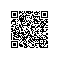# 整数划分算法原理与实现

整数划分问题是将一个正整数n拆成一组数连加并等于n的形式，且这组数中的最大加数不大于n。
如6的整数划分为

6
5 + 1
4 + 2, 4 + 1 + 1
3 + 3, 3 + 2 + 1, 3 + 1 + 1 + 1
2 + 2 + 2, 2 + 2 + 1 + 1, 2 + 1 + 1 + 1 + 1
1 + 1 + 1 + 1 + 1 + 1

共11种。下面介绍一种通过递归方法得到一个正整数的划分数。

递归函数的声明为 int split(int n, int m);其中n为要划分的正整数，m是划分中的最大加数(当m > n时，最大加数为n)，
1 当n = 1或m = 1时，split的值为1，可根据上例看出，只有一个划分1 或 1 + 1 + 1 + 1 + 1 + 1
可用程序表示为if(n == 1 || m == 1) return 1;

2 下面看一看m 和 n的关系。它们有三种关系
(1) m > n
在整数划分中实际上最大加数不能大于n，因此在这种情况可以等价为split(n, n);
可用程序表示为if(m > n) return split(n, n);
(2) m = n
这种情况可用递归表示为split(n, m - 1) + 1，从以上例子中可以看出，就是最大加
数为6和小于6的划分之和
用程序表示为if(m == n) return (split(n, m - 1) + 1);
(3) m < n
这是最一般的情况，在划分的大多数时都是这种情况。
从上例可以看出，设m = 4，那split(6, 4)的值是最大加数小于4划分数和整数2的划分数的和。
因此，split(n, m)可表示为split(n, m - 1) + split(n - m, m)

根据以上描述，可得源程序如下：

#include <stdio.h>

int split(int n, int m)
{

if(n < 1 || m < 1return 0;

if(n == 1 || m == 1return 1;

if(n < m) return split(n, n);

if(n == m) return (split(n, m - 1+ 1);

if(n > m) return (split(n, m - 1+ split((n - m), m));
}

int main()
{
printf(
"12的划分数: %d", split(1212));

return 0;
}

15

7 8

4 5 6

1 2 3 4 5

首先考虑一般的形式，设n为被划分的正整数，x为划分后最小的整数，如果n有一种划分，那么

这里还有一个问题，这个i的最大值是多少？不过有一点可以肯定，它一定比n小。我们可以做一个假设，

int split1(int n)
{

int i, j, m = 0, x, t1, t2;

// 在这里i + 1之所以变为i - 1，是因为i * (i - 1) / 2这个式子在下面多次用到，

// 为了避免重复计算，因此将这个值计算完后保存在t1中。并且将<= 号变为了<号。
for(i = 1; (t1 = i * (i - 1/ 2< n; i++
{
t2
= (n - t1);
x
=  t2 / i;

if(x <= 0break;

if((n - t1) % i == 0)
{
printf(
"%d ", x);

for(j = 1; j < i; j++)
printf(
"%d ", x + j);
printf(
"/n");
m
++;
}
}

return m;
}使用钉钉扫一扫加入圈子
+ 订阅